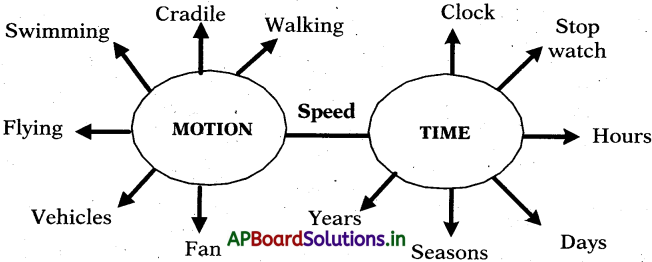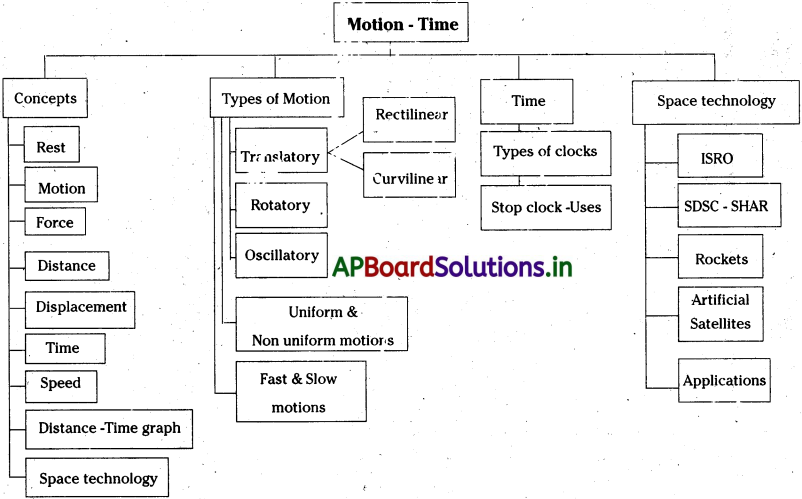# AP 7th Class Science Notes 5th Lesson Motion and Time

Students can go through AP Board 7th Class Science Notes 5th Lesson Motion and Time to understand and remember the concept easily.

## AP Board 7th Class Science Notes 5th Lesson Motion and Time

→ Motion is a common experience in our life.

→ Force makes an object to move or tend to move. It is required, to change the object from rest into motion, to change its direction and even to change from motion to rest.

→ The total length of a path taken by an object to reach one place from the other is called distance.

→ Primary unit of distance is centimeter. S.I units of distance is metre. But kilometre is used to measure large distances.

→ The change in position of an object is called displacement. It is the shortest distance between the starting and final positions.→ The measurable period between two incidents/ events is called time.

→ The basic unit of time is second. Larger units of time are minutes and hours.

→ An object is said to be in motion, if it changes its position with respect to surrounding and an object is said to be in rest, if it doesn’t change its position with respect to surroundings.

→ There are three, types of motion namely translatory motion, rotatory motion and oscil- iatory motion.

→ Speed of an object can be defined as the distance travelled by it in a unit time.

→ If all points of a moving object move through the same distance in same direction, then the motion is said to be translatory motion.

→ If a body in translatory motion moves along a straight, line then the motion is called rectilinear motion.

→ If a body in translatory motion move along a curved path, then the motion is called curvilinear motion.

→ If all the parts of a moving body follow a curved path with respect to a fixed centre or axis of rotation, it is said to be in rotatory motion.

→ Sometimes, an object may possess both translatory, and rotatory motions. For ex¬ample cycle tyre moving in a straight line.

→ The ‘to and fro’ motion of an object about a fixed point that always following the same path is called oscillatory or vibratory motion.

→ If a body covers equal distances in equal intervals of time, it is said to be in uniform motion.→ If a body covers unequal distances in equal intervals of time, it is said to be in non¬uniform motion.

→ The distance travelled by an object in a. given interval of time can help us to decide whether it is faster or slower.

→ Speedometer helps us to know the speed of a vehicle.

→ Units of speed is meter per second (m/s)or kilometre per hour (Km/h)

→ 1 Km / h = 5/18 m/s

→ Rockets are devices that produce force or push needed to move an object forward. Rockets are used to launch space crafts and satellites. They are also used to shoot missiles.

→ ISRO : (Indian Space Research Organisation) formed in 1969.

→ SLV : Satellite Launch Vehicle.

→ ASLV : Augmented Satellite Launch Vehicle.

→ PSLV: Polar Satellite Launch Vehicle.

→ GSLV: Geosynchronous Satellite Launch Vehicle.

→ SDSC : Satish Dhawan Space Centre.

→ SHAR : Sriharikota High Altitude Range.

→ DOS : Department Of Space.

→ An artificial satellite is a manmade object, Iavnched to revolve around the Earth.

→ Artificial satellites are used in communication and navigation.

→ Motion : If an object changes its position with respect to its surroundings, it is said to be in motion.

→ Rest : An object is said to be at rest if there is no change in its position with respect to its surroundings.

→ Force : Force is a push, pull, or hit on any object that brings a change in its state of motion.→ Distance : The total length of the path travelled by an object between two places is called distance.

→ Displacement : The change in position of an object is called displacement. It is the shortest distance between the starting and final positions.

→ Time : The measurable period between two incidents/ events is called time.

→ Translatory motion : If all points of a moving object move through the same distance in same direction, then the motion is said to be translatory motion.

→ Rotatory Motion : If all the parts of a moving body follow a curved path with respect to a fixed centre or .axis of rotation, it is said to be in rotatory motion.

→ Axis of Rotation : The imaginary line passing through the fixed centre around which the parts of the objects move in a curved path during rotatory motion is called axis of rotation.

→ Oscillatory Motion : The ‘to and fro’ motion of an object about a fixed point that always following the same path is called oscillatory or vibratory motion.

→ Uniform motion : If a body covers equal distances in equal intervals of time, it is said to be in uniform motion.

→ Non uniform motion : If a body covers unequal distances in equal intervals of time, it is said to be in non-uniform motion.→ Speed : The distance travelled by an object in a unit time is called its speed.

→ Speedometer : Speedometer is an instrument that indicates the speed of a vehicle.

→ Odometer : An odometer is an instrument used for measuring the distance traveled by a vehicle.

→ Average Speed : Average speed is the ratio of total distance covered and total time taken by the body to cover the distance.

→ Rockets : Rockets are devices that produce force or push needed to move an object forward. They are used to launch spate crafts and satellites.

→ Artificial Satellite : An artificial satellite is a manmade object, launched to revolve around the Earth.